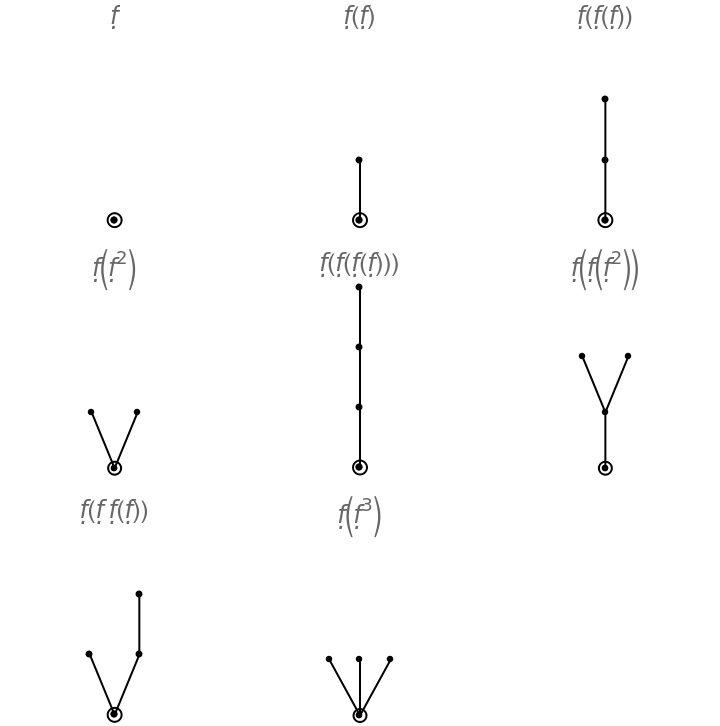#Function Repository Resource:

# ButcherPlot

Plot order condition trees for Runge–Kutta method

Contributed by: Wolfram Research
 ResourceFunction["ButcherPlot"][tree] gives a plot of the Butcher tree tree. ResourceFunction["ButcherPlot"][{tree1,tree2,…}] gives an array of plots of the treei.

## Details and Options

Rooted trees in ResourceFunction["ButcherPlot"][] correspond to the elementary differentials appearing in the Taylor expansion of the system of differential equations, and branching of a sub-tree corresponds to exponentiation in the differential equation.
In Butcher plots, roots are denoted by circles and the number of vertices in a tree correspond to the order of the tree.
The following options can be given:
 "ButcherPlotColumns" Automatic number of columns in an array of plots "ButcherPlotNodeSize" 1 scaling factor for the nodes in the trees "ButcherPlotRootSize" 1 scaling factor for highlighting roots "ButcherPlotLabel" { } list of plot labels

## Examples

### Basic Examples

Generate and plot trees for any Runge–Kutta method of order 3:

 In:=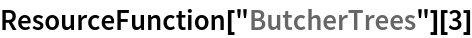Out=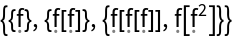In:=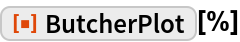Out=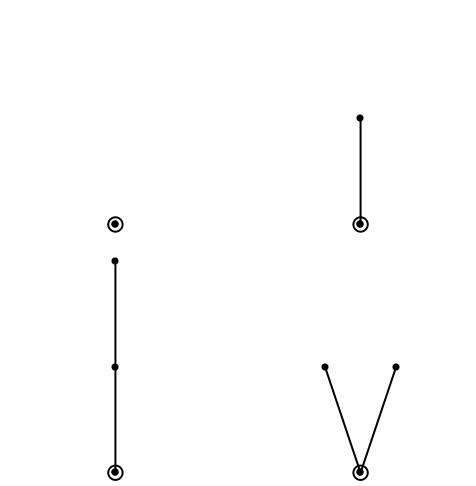### Scope

Plot a Butcher tree:

 In:=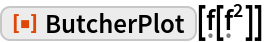Out=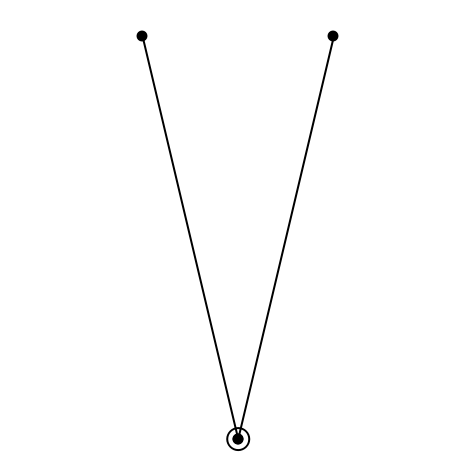Plot several trees:

 In:=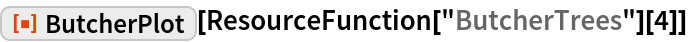Out=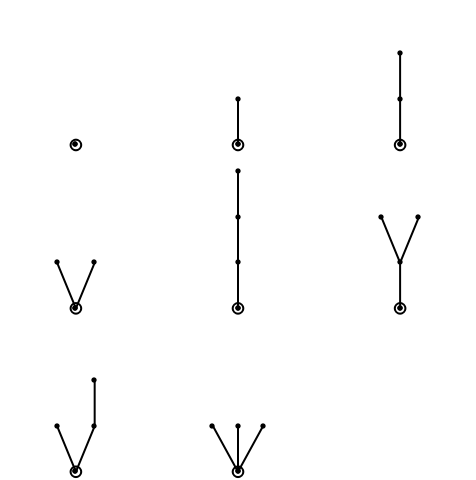### Options

#### ButcherPlotColumns

Plot trees in a single column:

 In:=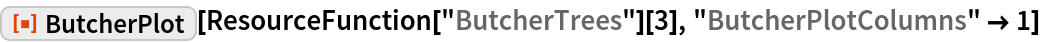Out=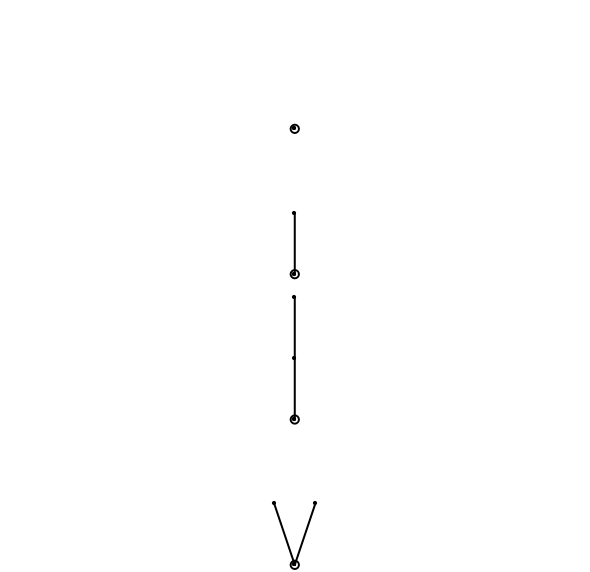#### ButcherPlotNodeSize

Increase the size of nodes:

 In:=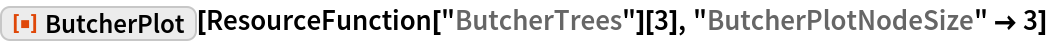Out=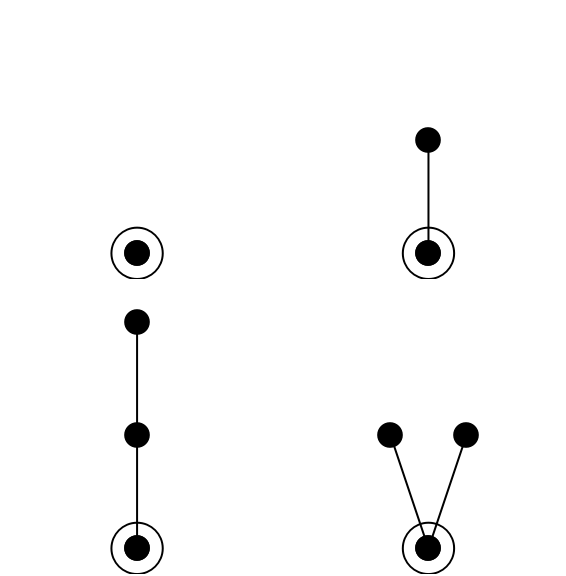#### ButcherPlotRootSize

Highlight roots with larger circles:

 In:=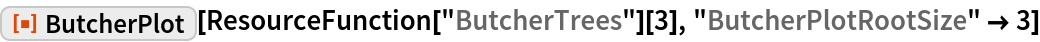Out=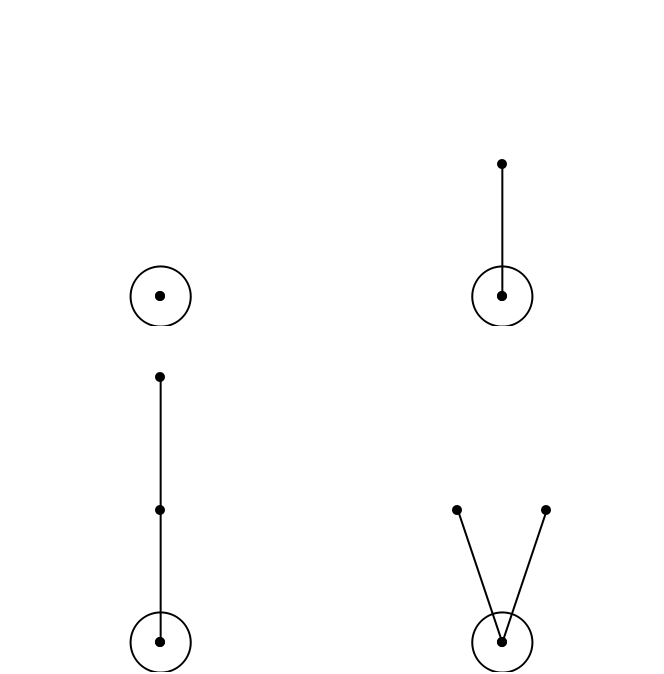#### ButcherPlotLabel

Label trees:

 In:=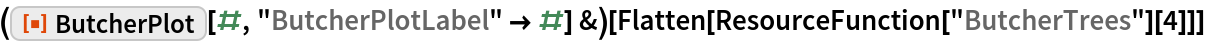Out=